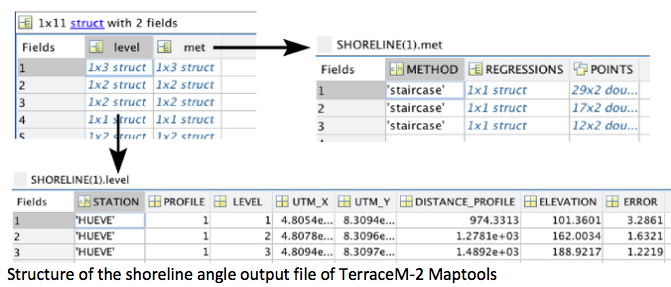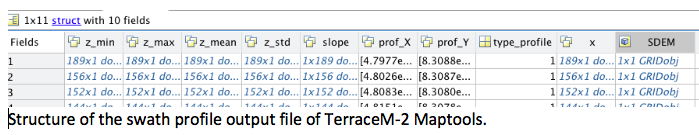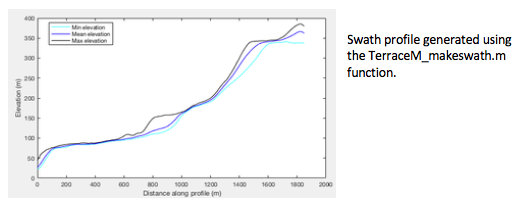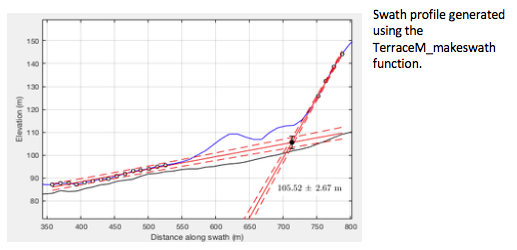| Home |

## BLOG

Sunday 28 July 2019 at 09:06 am.

This section presents some examples of the command line capabilities of TerraceM outside the Graphic user interface.

TerraceM-2 Maptools creates several Matlab® files used for data exchange between the other TerraceM-2 modules. These files can be called independently to create customized plots. In this example, we load the main output files containing the shoreline angles and swath profiles.

The shoreline-angle file (.mat) is a structure containing several fields that define: a) method (met) used to map the shoreline angles, including the linear regression coefficients and points used for mapping, and b) the shoreline angle level (level), which includes the profile, elevation, error, map coordinates, location along profile and the station or mapping site name.## Displaying swath profiles and shorelines angles mapped in TerraceM-2

This excercise follows the previous example.

Here we present an example plotting swath profiles and shoreline angles using the output files of TerraceM-2 Maptools.

i=2; %profile number

j=1; %marine terrace level

%these values can be eventually looped to plot all mapped shoreline angles

%extract the linear regressions for platform and cliff

cliff=SHORELINE(i).met(j).REGRESSIONS.cliff;

plat=SHORELINE(i).met(j).REGRESSIONS.plat;

points=SHORELINE(i).met(j).POINTS;

%extract the shoreline angle

SH(1)=SHORELINE(i).level(j).DISTANCE_PROFILE;

SH(2)=SHORELINE(i).level(j).ELEVATION;

SH(3)=SHORELINE(i).level(j).ERROR;

%plot the result

figure

hold on; plot(swath(i).x,swath(i).z_max,'-k',swath(i).x,swath(i).z_min,...

'-k',swath(i).x,swath(i).z_mean,'-k');%plot the swath profile

plot(cliff(:,1),cliff(:,2),'--r'); plot(cliff(:,1),cliff(:,4),'--r');%cliff points

plot(cliff(:,1),cliff(:,3),'-r');plot(plat(:,1),plat(:,2),'--r');%platform points

plot(plat(:,1),plat(:,4),'--r');plot(plat(:,1),plat(:,3),'-r');%regressions

errorbar(SH(1),SH(2),SH(3),'ok'); plot(SH(1),SH(2),'ok',...  %shoreline angle

'MarkerFaceColor','r','Markersize',6);

xlabel('Distance along profile (m)');ylabel('Elevation (m)');%axis labels

## Creating swath profiles from shapefile polygons outside the GUI environment

Swath profiles can be generated using the TerraceM_makeswath.m function and Topotoolbox. The inputs are a DEM in geotiff (.tif) or Esri grid format (.asc) and a shapefile containing the swath profiles boxes, which may be created using the rectangle tool. The output is a structure containing the distance, minimum, mean, maximum and slope of a swath profile.

%Add path to topotoolbox folder, TerraceM-2 Maptools, and dem and shapefile.

Box=shaperead('Huevo_clip.shp'); %Shapefile containing the profiles boxes

DEM=GRIDobj('DEM_huevo.tif'); %DEM generated using Topotoolbox

profnum=2; %Profile number to process

%Create swath function

swat=TerraceM_makeswath(DEM,Box,profnum);

%Display swath profile

figure

plot(swat.x,swat.z_min,'-c',swat.x,swat.z_mean,'-b',swat.x,swat.z_max,'-k');%plot the swath lines

xlabel('Distance along profile (m)'); ylabel('Elevation (m)');

legend('Min elevation','Mean elevation','Max elevation')## Mapping shoreline angles outside the GUI environment

This excercise follows the swath profile plot excercise presented before.

We can directly map shoreline angles calling the function TerraceM_staircase.m, which displays an interactive window to map the shoreline angle by selecting the paleo-platform and paleo-cliff. Outputs include the shoreline angle elevation and its associated error (shoreline), the points used to fit the linear regressions (points), and the linear regression coefficients and 95% confidence bounds (regr).

%Mapping a single shoreline angle

[shoreline,points,regr]=TerraceM_staircase(swat);More scripts related to LEM and DIM are comming soon...